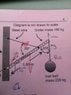# Torque and Forces Question (With a Diagram)

• Siann122
In summary, the ball is hanging from a wire and has a weight of 220kg. The rod has a mass of 180kg and is pivoted at a point 1.6m from the wire. The tension in the wire is 5390N. The reaction force from the pivot is calculated to be 5390N.

## Homework Statement

https://www.physicsforums.com/attachment.php?attachmentid=40303&stc=1&d=1319521828

An iron ball of mass 220kg is suspended from the end of a rigid steel girder. The girder has a mass of 180kg and a length of 2.4m. The girder is pivoted to a rigid upright. A steel wire is attached 1.6m along the girder. It holds it in equilibrium with angles between component as shown in the diagram.

a). Identify all the forces acting on the girder by drawing them on the diagram.
b). Demonstrate by calculation that the tension in the steel wire is 5390N
c). If the tension in the steel wire is 5390N, calculate the magnitude and direction of the reaction force from the pivot acting on the steel girder.

F = ma
T = rF
Ʃ = 0

## The Attempt at a Solution

I have absolutely no idea how to even attempt this question, I just don't know where to start.

#### Attachments

•New Image.JPG
10.3 KB · Views: 665
Draw a free body diagram of the rod. Label all the forces acting on it and break them into x and y components.

Sum the forces in the x and y directions and know they must be equal to zero.

Next sum the torques.

aftershock said:
Draw a free body diagram of the rod.

The diagram drawing itself is confusing me. Does the ball and mg go in the same place (the center of gravity on the rod) or does a separate arrow need to be drawn in for the ball?

aftershock said:
Draw a free body diagram of the rod. Label all the forces acting on it and break them into x and y components.

Sum the forces in the x and y directions and know they must be equal to zero.

Next sum the torques.

Great solution! :)

Siann122 said:
The diagram drawing itself is confusing me. Does the ball and mg go in the same place (the center of gravity on the rod) or does a separate arrow need to be drawn in for the ball?

Draw a free body diagram of the rod only. The ball is not part of this. What is part of this is the tension in the rope where the ball is hanging, that is equal to the balls weight. So you have the weight of the ball acting where the rope is and then you have mg of the rod acting at the rods center of gravity which is half the length of the rod (we have to assume the density is constant if it doesn't say that otherwise it becomes a little more complicated).

Coto said:
Great solution! :)

lol was that sarcastic? Should I have added anything else?